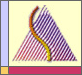# SCIENTIFIC PROGRAMS AND ACTIVITIESJune  8, 2023##June 15-27, 2009 Summer School June 28-July 3, 2009 Conference in Geometric Representation Theory and Extended Affine Lie Algebras at the University of Ottawa, Ontario, Canada

### Week 1:

Joel Kamnitzer (University of Toronto)
Introduction to geometric representation theory

As an introduction to geometric representation theory, I will present three different constructions of the irreducible representations of GL_n. All three constructions involve the geometry of the flag variety and related varieties. We will begin with the most ``classical'' construction -- the Borel-Weil theorem, which constructs representations as sections of line bundles on the flag variety. Next, we will consider Ginzburg's construction of representations using the homology of partial Springer fibres. Finally, we will look at the geometric Satake correspondence, where we realize representations using the intersection homology of orbit closures in the affine Grassmannian. All our constructions generalize to all complex reductive groups, but we will focus on GL_n in order to simplify the notation and to make the geometry more transparent.

It would be helpful if the participants know the representation theory of GL_n (classification of representations by highest weight) and some of the geometry of the flag variety, but these topics will be reviewed during the course.

Seaok-Jin Kang (Seoul National University)
Introduction to quantum groups and crystals

In this course, we will give an introduction to the theory of quantum groups and crystal bases. We will start with the basic theory of Kac-Moody algebras and their quantum deformation. We then move on to the crystal basis theory including tensor product rule, abstract crystals and global bases. As an illustration, the crystal bases for quantum general linear algebras will be realized as the crystals consisting of semistandard Young tableaux. Our main objective is to study the perfect crystals for quantum affine algebras. Using the fundamental crystal isomorphism theorem, we will give realizations of irreducible highest weight crystals in terms of Kyoto paths. Finally, we will discuss combinatorics of Young walls. The Young walls consist of colored blocks with various shapes and can be regarded as generalizations of Young diagrams. The irreducible highest weight crystals for classical quantum affine algebras will be realized as the affine crystals consisting of reduced Young walls.

Prerequisites: I will define and review Kac-Moody algebras and their representation theory. I will assume that students are familiar with the materials in the 1st year graduate algebra course, e.g. rings, modules, tensor product, but no more than that.

Erhard Neher (University of Ottawa)
Affine, toroidal and extended affine Lie algebras

The course will give an introduction to generalizations of affine Lie algebras. The focus will be on extended affine Lie algebras, whose structure theory will be presented: root systems, construction of extended affine Lie algebras in terms of generalized loop algebras, the so-called Lie tori. To illustrate the general theory we will present many examples, like toroidal algebras and extended affine Lie algebras associated to quantum tori and multiloop algebras.

Prerequisites: Basic theory of simple finite-dimensional Lie algebras. Some understanding of affine Lie algebras will be helpful, but not
required.

### WEEK 2:

Weiqiang Wang (University of Virginia)
Nilpotent orbits and W algebras

In recent years, the (finite) W algebras associated to a simple Lie algebra \$g\$ and a nilpotent element of \$g\$ (which can be traced back to Kostant and Lynch) have been studied intensively by many people from different viewpoints including Premet, Skryabin, Brundan, Kleshchev, Gan, Ginzburg, an others. This mini-course is aimed at non-experts with a good background on basic Lie theory. We shall present some basic constructions, connections, and applications of finite W algebras over the complex field, with particular focus on type A, such
as:

-- various equivalent definitions of W algebras.
-- independence of W algebras on good gradings.
-- Yangians and W algebras.
-- equivalence between module category of W algebras and Whittaker category of g-modules.
-- higher level Schur duality between W algebras and cyclotomic Hecke algebras
-- (if time permits), generalizations of W algebras to the affine, super, modular settings, etc.

Alistair Savage (University of Ottawa)
Geometric realizations of crystals

This course will follow up on and combine ideas presented in the first week courses by Kang and Kamnitzer. In particular, Kamnitzer will discuss various varieties used in geometric realizations of representations of certain Lie algebras. In this course, we will see how one can use these varieties to obtain geometric realizations of the crystals (which are combinatorial objects) associated to these representations and introduced in the course by Kang. A typical construction will see the vertex set of a crystal appearing as the set of irreducible components of certain varieties, with the crystal operators being realized as natural geometric operations.

Vyjayanthi Chari (UC Riverside)
Representation theory of affine and toroidal Lie algebras

The plan of lectures is as follows.
Lecture 1: Representations of finite--dimensional simple Lie algerbas, the Harishchandra homomorphism, central characters.
Lecture 2. Positive level representations of affine Lie algebras.
Lectures 3 and 4. Level zero representations of affine Lie algebras.
Lecture 5. The higher dimensional case.